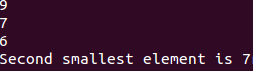# Programming Videos

## Search

### How to Find Second Smallest Element in an Array

Write a program to find the second smallest element in an array using sorting.
The element in an array is non-repeating.

There are many approaches to solved this problem.

To solve this problem, i use approach which involves sorting of an array

1. Sort the array. For sorting you can use bubble sort, quicksort etc.

2. Once the array is sorted you can easily pick the second smallest element in an array which is the second index of an array a.

Find second smallest element in an array without using sorting
```
```
```
#include<stdio.h>
```
```int main(){

int a,size,i,j;
```
```  printf("Enter the size of the array: ");
scanf("%d",&size);
printf("Enter %d elements in to the array: ", size);
```
```    for(i=0;i<size;i++)
scanf("%d",&a[i]);

// Sort the array using bubble sort

for(i=0;i<size;i++)
{
int temp=0;
for(j=i+1;j<size;j++)
{
if(a[i]>a[j])
{
temp = a[i];
a[i] = a[j];
a[j] = temp;
}
}
}

// Now the array is sorted you can pick the second element by a

printf("Second smallest element is",a);

}
```
```

Output-

Enter the size of the array : 3

Enter 3 elements into the array```